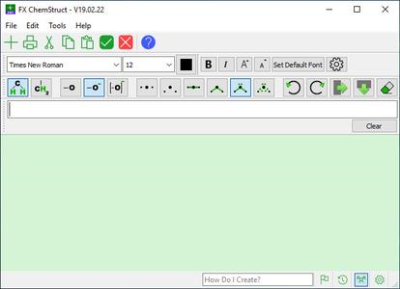• Login:
• Password:

# FX Science Tools 19.02.26

Error: king |complaint11-03-2019, 12:56FX Science Tools 19.02.26 | 38.6 Mb

FX Science Tools provide chemistry and physics teachers with highly efficient means of creating equations and chemical structures. Just type and create.

FX Chem FX Chem makes writing chemical equations dead simple.

FX Science Tools provide chemistry and physics teachers with highly efficient means of creating equations and chemical structures. Just type and create.
Type Chemistry - FX Chem
FX Chem FX Chem makes writing chemical equations dead simple. You just type and FX Chem knows enough about chemistry to put all of the components of the equation into the right spots. FX Chem can even produce nuclear chemistry equations - something that is very difficult in Word.
Type Chemical Structures - FX ChemStruct
FX ChemStruct FX ChemStruct does to organic structures what FX Chem does for chemical equations. Just type the structural formula of a compound and FX ChemStruct will draw a structural diagram. No "drawing" required.
Type Physics - FX Equation
FX Equation FX Equation is a totally different way of entering equations. No other program can rival FX Equation's easy, high speed creation of secondary science equations. FX Equation is for anyone who gets frustrated with their current equation editor.
Windows 7, 8/8.1, 10
-
DOWNLOAD
(Buy premium account for maximum speed and resuming ability)